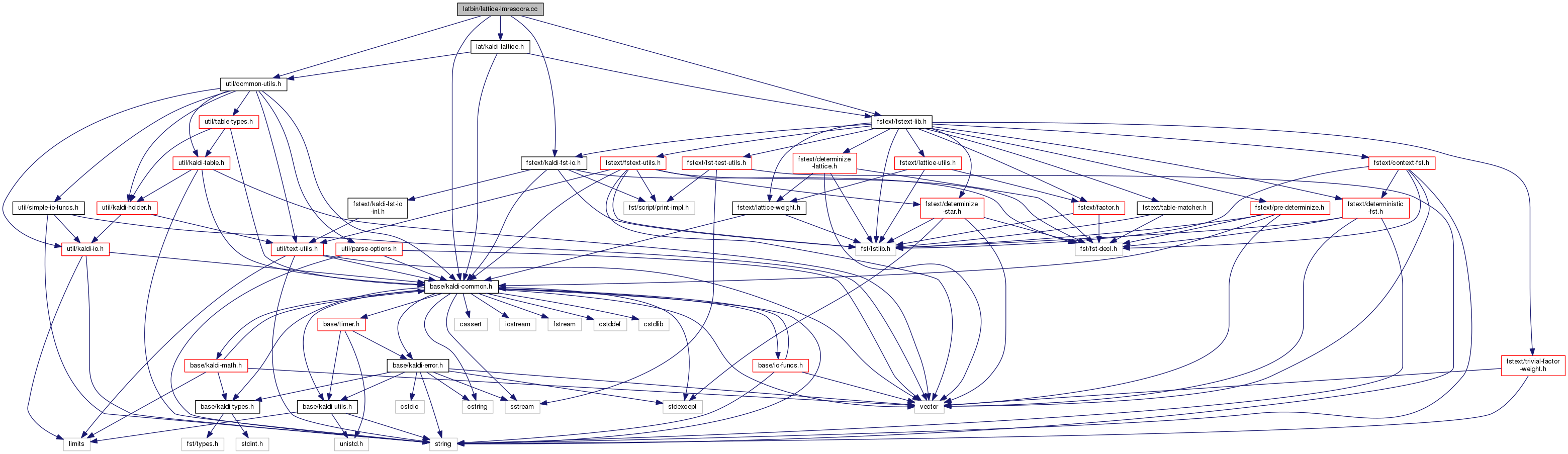lattice-lmrescore.cc File Reference
`#include "base/kaldi-common.h"`
`#include "util/common-utils.h"`
`#include "fstext/fstext-lib.h"`
`#include "fstext/kaldi-fst-io.h"`
`#include "lat/kaldi-lattice.h"`
Include dependency graph for lattice-lmrescore.cc:Go to the source code of this file.

## Functions

int main (int argc, char *argv[])

## ◆ main()

 int main ( int argc, char * argv[] )

Definition at line 28 of file lattice-lmrescore.cc.

28  {
29  try {
30  using namespace kaldi;
31  typedef kaldi::int32 int32;
32  typedef kaldi::int64 int64;
33  using fst::SymbolTable;
34  using fst::VectorFst;
35  using fst::StdArc;
37
38  const char *usage =
39  "Add lm_scale * [cost of best path through LM FST] to graph-cost of\n"
40  "paths through lattice. Does this by composing with LM FST, then\n"
41  "lattice-determinizing (it has to negate weights first if lm_scale<0)\n"
42  "Usage: lattice-lmrescore [options] <lattice-rspecifier> <lm-fst-in> <lattice-wspecifier>\n"
43  " e.g.: lattice-lmrescore --lm-scale=-1.0 ark:in.lats 'fstproject --project_output=true data/lang/G.fst|' ark:out.lats\n";
44
45  ParseOptions po(usage);
46  BaseFloat lm_scale = 1.0;
47  int32 num_states_cache = 50000;
48
49  po.Register("lm-scale", &lm_scale, "Scaling factor for language model costs; frequently 1.0 or -1.0");
50  po.Register("num-states-cache", &num_states_cache,
51  "Number of states we cache when mapping LM FST to lattice type. "
52  "More -> more memory but faster.");
53
55
56  if (po.NumArgs() != 3) {
57  po.PrintUsage();
58  exit(1);
59  }
60
61  std::string lats_rspecifier = po.GetArg(1),
62  fst_rxfilename = po.GetArg(2),
63  lats_wspecifier = po.GetArg(3);
64
65
66
67  VectorFst<StdArc> *std_lm_fst = ReadFstKaldi(fst_rxfilename);
68  if (std_lm_fst->Properties(fst::kILabelSorted, true) == 0) {
69  // Make sure LM is sorted on ilabel.
70  fst::ILabelCompare<StdArc> ilabel_comp;
71  fst::ArcSort(std_lm_fst, ilabel_comp);
72  }
73
74  // mapped_fst is the LM fst interpreted using the LatticeWeight semiring,
75  // with all the cost on the first member of the pair (since it's a graph
76  // weight).
77  fst::CacheOptions cache_opts(true, num_states_cache);
78  fst::MapFstOptions mapfst_opts(cache_opts);
80  fst::MapFst<StdArc, LatticeArc, fst::StdToLatticeMapper<BaseFloat> >
81  lm_fst(*std_lm_fst, mapper, mapfst_opts);
82  delete std_lm_fst;
83
84  // The next fifteen or so lines are a kind of optimization and
85  // can be ignored if you just want to understand what is going on.
86  // Change the options for TableCompose to match the input
87  // (because it's the arcs of the LM FST we want to do lookup
88  // on).
90  true, fst::SEQUENCE_FILTER,
91  fst::MATCH_INPUT);
92
93  // The following is an optimization for the TableCompose
94  // composition: it stores certain tables that enable fast
95  // lookup of arcs during composition.
96  fst::TableComposeCache<fst::Fst<LatticeArc> > lm_compose_cache(compose_opts);
97
98  // Read as regular lattice-- this is the form we need it in for efficient
99  // composition and determinization.
101
102  // Write as compact lattice.
103  CompactLatticeWriter compact_lattice_writer(lats_wspecifier);
104
105  int32 n_done = 0, n_fail = 0;
106
108  std::string key = lattice_reader.Key();
109  Lattice lat = lattice_reader.Value();
111  if (lm_scale != 0.0) {
112  // Only need to modify it if LM scale nonzero.
113  // Before composing with the LM FST, we scale the lattice weights
114  // by the inverse of "lm_scale". We'll later scale by "lm_scale".
115  // We do it this way so we can determinize and it will give the
116  // right effect (taking the "best path" through the LM) regardless
117  // of the sign of lm_scale.
118  fst::ScaleLattice(fst::GraphLatticeScale(1.0 / lm_scale), &lat);
119  ArcSort(&lat, fst::OLabelCompare<LatticeArc>());
120
121  Lattice composed_lat;
122  // Could just do, more simply: Compose(lat, lm_fst, &composed_lat);
123  // and not have lm_compose_cache at all.
124  // The command below is faster, though; it's constant not
125  // logarithmic in vocab size.
126  TableCompose(lat, lm_fst, &composed_lat, &lm_compose_cache);
127
128  Invert(&composed_lat); // make it so word labels are on the input.
129  CompactLattice determinized_lat;
130  DeterminizeLattice(composed_lat, &determinized_lat);
131  fst::ScaleLattice(fst::GraphLatticeScale(lm_scale), &determinized_lat);
132  if (determinized_lat.Start() == fst::kNoStateId) {
133  KALDI_WARN << "Empty lattice for utterance " << key << " (incompatible LM?)";
134  n_fail++;
135  } else {
136  compact_lattice_writer.Write(key, determinized_lat);
137  n_done++;
138  }
139  } else {
140  // zero scale so nothing to do.
141  n_done++;
142  CompactLattice compact_lat;
143  ConvertLattice(lat, &compact_lat);
144  compact_lattice_writer.Write(key, compact_lat);
145  }
146  }
147
148  KALDI_LOG << "Done " << n_done << " lattices, failed for " << n_fail;
149  return (n_done != 0 ? 0 : 1);
150  } catch(const std::exception &e) {
151  std::cerr << e.what();
152  return -1;
153  }
154 }
This code computes Goodness of Pronunciation (GOP) and extracts phone-level pronunciation feature for...
Definition: chain.dox:20
void TableCompose(const Fst< Arc > &ifst1, const Fst< Arc > &ifst2, MutableFst< Arc > *ofst, const TableComposeOptions &opts=TableComposeOptions())
fst::StdArc StdArc
A templated class for writing objects to an archive or script file; see The Table concept...
Definition: kaldi-table.h:368
kaldi::int32 int32
TableComposeCache lets us do multiple compositions while caching the same matcher.
float BaseFloat
Definition: kaldi-types.h:29
The class ParseOptions is for parsing command-line options; see Parsing command-line options for more...
Definition: parse-options.h:36
void ScaleLattice(const std::vector< std::vector< ScaleFloat > > &scale, MutableFst< ArcTpl< Weight > > *fst)
Scales the pairs of weights in LatticeWeight or CompactLatticeWeight by viewing the pair (a...
void ConvertLattice(const ExpandedFst< ArcTpl< Weight > > &ifst, MutableFst< ArcTpl< CompactLatticeWeightTpl< Weight, Int > > > *ofst, bool invert)
Convert lattice from a normal FST to a CompactLattice FST.
A templated class for reading objects sequentially from an archive or script file; see The Table conc...
Definition: kaldi-table.h:287
fst::VectorFst< LatticeArc > Lattice
Definition: kaldi-lattice.h:44
TableMatcher is a matcher specialized for the case where the output side of the left FST always has e...
Definition: table-matcher.h:42
#define KALDI_WARN
Definition: kaldi-error.h:150
fst::VectorFst< CompactLatticeArc > CompactLattice
Definition: kaldi-lattice.h:46
std::vector< std::vector< double > > GraphLatticeScale(double lmwt)
Class StdToLatticeMapper maps a normal arc (StdArc) to a LatticeArc by putting the StdArc weight as t...
void ReadFstKaldi(std::istream &is, bool binary, VectorFst< Arc > *fst)
bool DeterminizeLattice(const Fst< ArcTpl< Weight > > &ifst, MutableFst< ArcTpl< Weight > > *ofst, DeterminizeLatticeOptions opts, bool *debug_ptr)
This function implements the normal version of DeterminizeLattice, in which the output strings are re...
#define KALDI_LOG
Definition: kaldi-error.h:153